# TRANSLATIONS OF TRIGONOMETRIC GRAPHS 12 8 SINE OR

• Slides: 10
Download presentation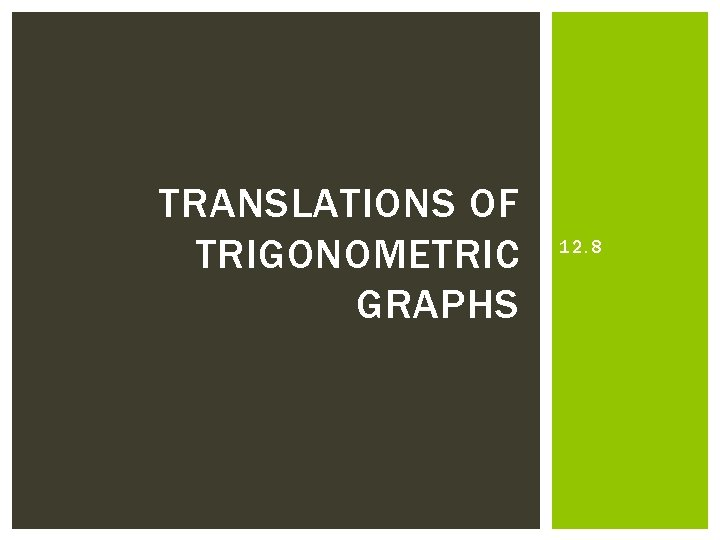TRANSLATIONS OF TRIGONOMETRIC GRAPHS 12. 8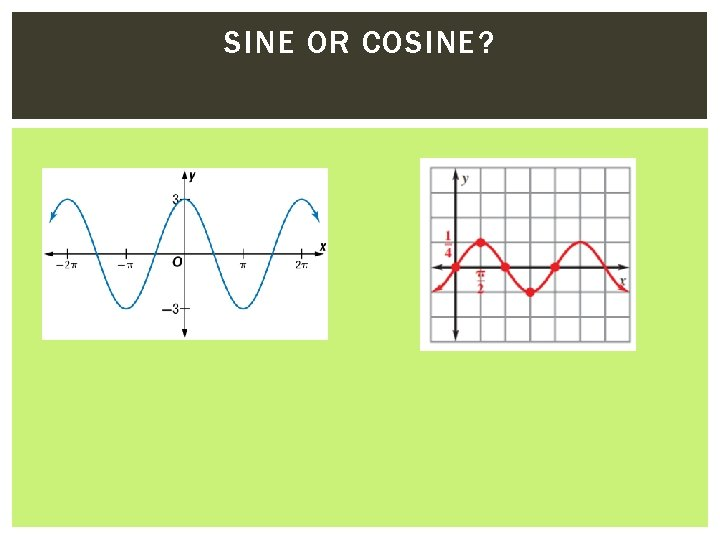SINE OR COSINE?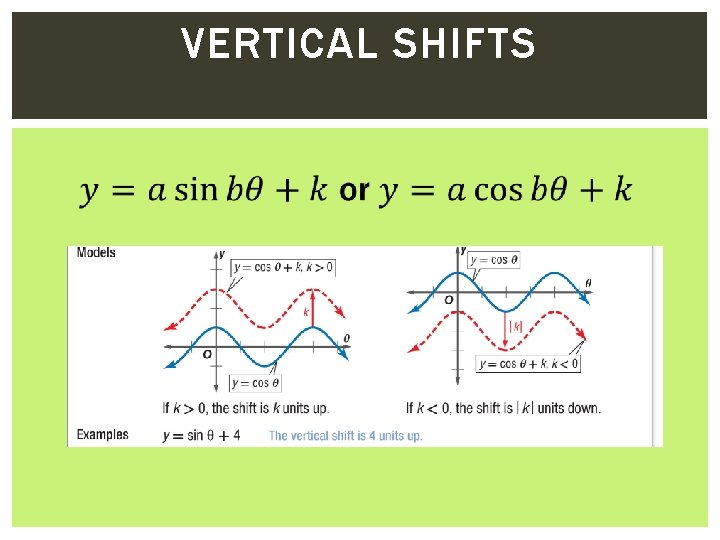VERTICAL SHIFTS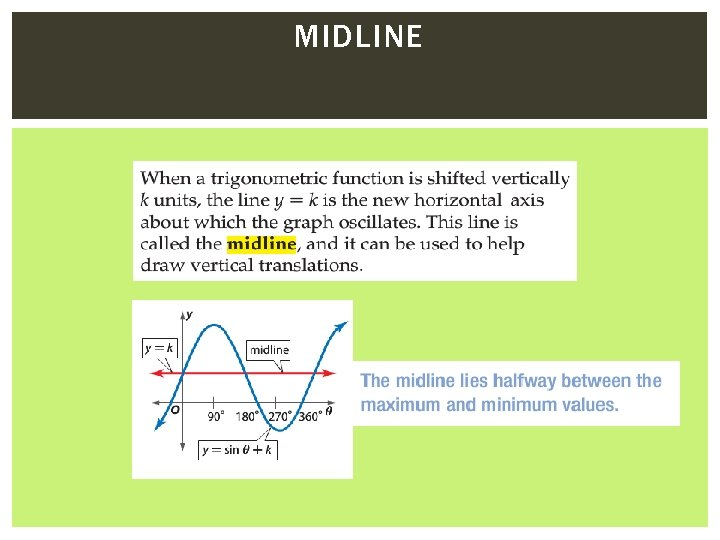MIDLINEEXAMPLE 1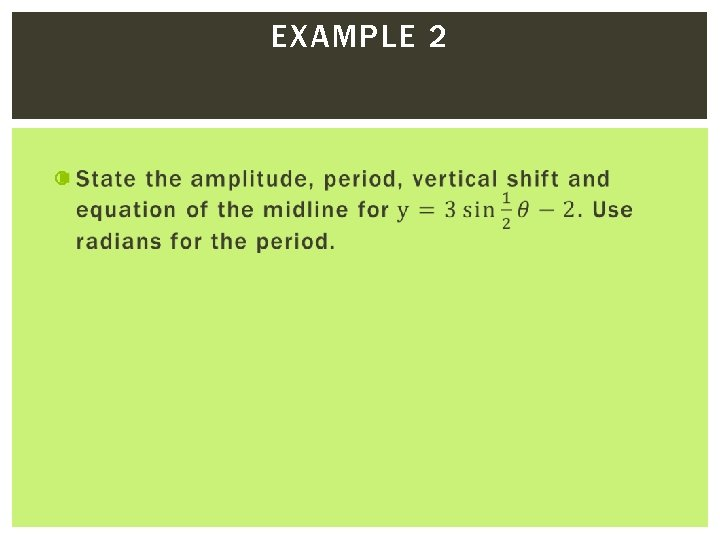EXAMPLE 2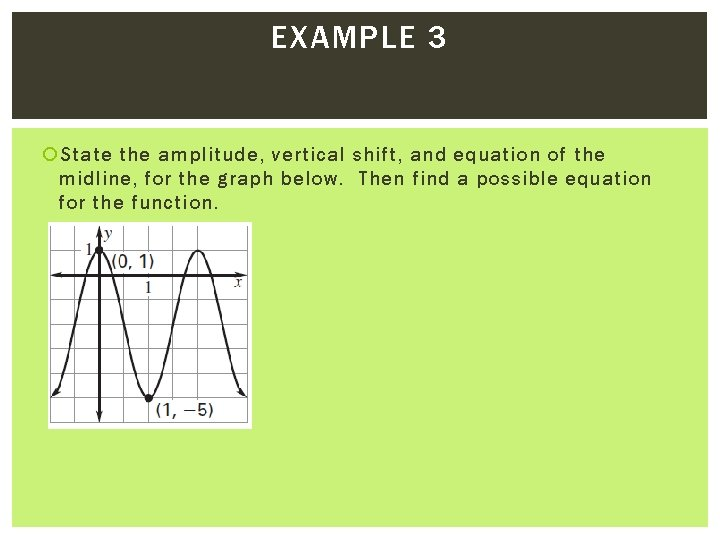EXAMPLE 3 State the amplitude, vertical shift, and equation of the midline, for the graph below. Then find a possible equation for the function.EXAMPLE 4 State the amplitude, period, vertical shift, and equation of the midline, for the graph below. Then find a possible equation for the function.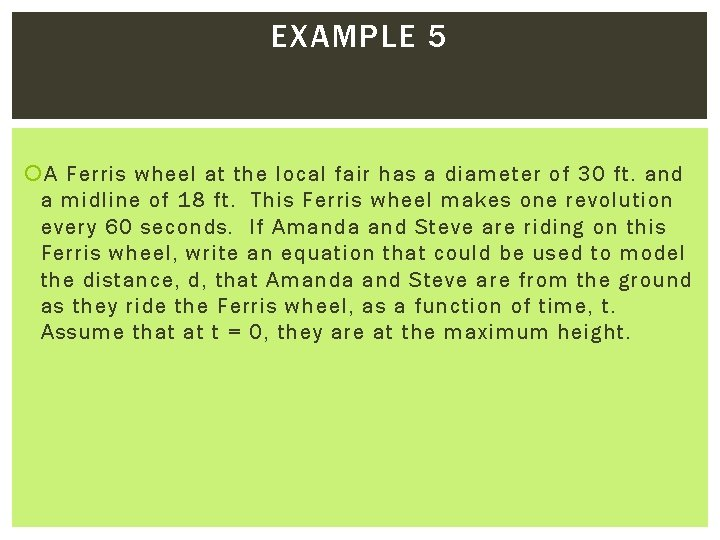EXAMPLE 5 A Ferris wheel at the local fair has a diameter of 30 ft. and a midline of 18 ft. This Ferris wheel makes one revolution every 60 seconds. If Amanda and Steve are riding on this Ferris wheel, write an equation that could be used to model the distance, d, that Amanda and Steve are from the ground as they ride the Ferris wheel, as a function of time, t. Assume that at t = 0, they are at the maximum height.EXAMPLE 6 The monthly normal temperatures for San Diego, CA, can oscillate between a maximum of 73 degrees and a minimum of 57 degrees. The months are represented by the integers 1, 2, , 12 and the temperature is in degrees Fahrenheit. Let y be the temperature and x be the integer representing the month. Write a function that represents the monthly normal temperatures for San Diego. Assume that the temperature is the median value at t = 0.